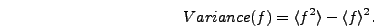Next: The Scale Factor and Up: Output Previous: Run Parameters

# Field Means and Variances

The function meansvars() calculates the means and variances of all output fields. The means are simply the sum of the field at each gridpoint divided by the total number of gridpoints. The variance is calculated as(5.1)

The means and variances are stored in files called means_ext and variance_ext. Each of these fields contains one column for the time and one column each for the output fields.

The meansvars() function is also where the program checks for runaway field behavior. If the evolution becomes unstable (usually due to using too large a time step) the fields can start growing exponentially. In this case it will generally not take many time steps before the field values exceed the maximum storage possible on the computer, and the values are then simply stored as non-numerical. The program checks for such an occurence by verifying that the average is numerical. If this test fails then the program will print a warning message, call output_parameters() to record the elapsed time for the run, flush the output buffer for means and variances, and abort. Usually the solution in such a case is to reduce the time step and rerun.

Note that if you choose not to output means and variances then this error checking won't occur. In practice, though, it is almost invariably useful to have these quantities for at least one of the output fields, so we recommend leaving this output field on for all runs.Next: The Scale Factor and Up: Output Previous: Run Parameters